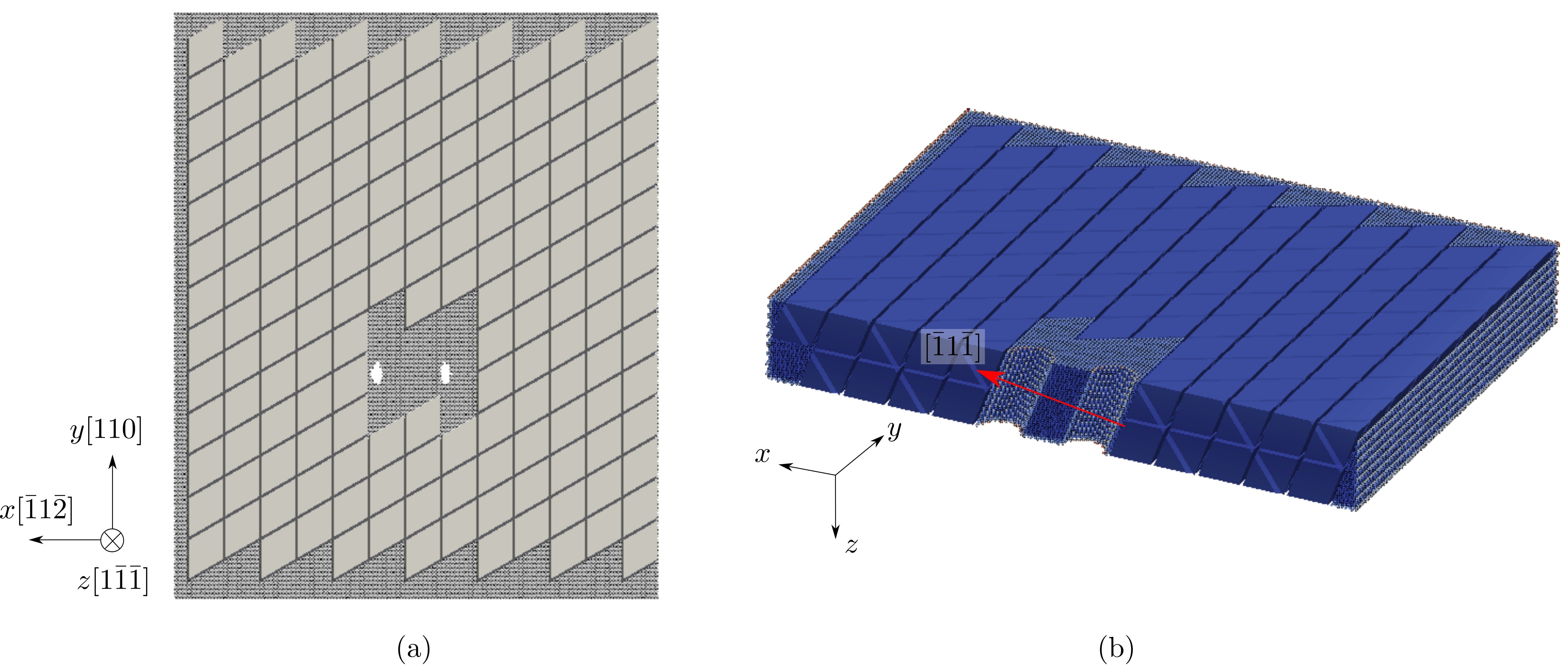# Dislocation multiplication from a Frank-Read source

FCC Al, Mishin EAM potential, 2197 atoms per element in the coarse-grained domain. Results using larger models are published in Xu et al., 2016 and Xu et al., 2016.

In the figure below, two cylindrical holes are carved out to serve as the Frank-Read source. The atoms and elements in figure (b) are colored by the atomic and nodal energy, respectively, and are sliced on the $xz$ plane to highlight the holes. In the hybrid simulation, an edge dislocation is first created between the two holes; then subject to a $\gamma_{zy}$ simple shear strain, it bows out and form a dislocation loop, leaving behind another edge dislocation segment between the two holes.The movie below and the log file are produced using the input fileand rendered by OVITO: